## 3.7  Exponential Divergence

Hénon and Heiles discovered that the chaotic trajectories had remarkable sensitivity to small changes in initial conditions -- initially nearby chaotic trajectories separate roughly exponentially with time. On the other hand, regular trajectories do not exhibit this sensitivity -- initially nearby regular trajectories separate roughly linearly with time.

Consider the evolution of two initially nearby trajectories for the Hénon-Heiles problem, with energy E = 1/8. Let d(t) be the usual Euclidean distance in the x, y, px, py space between the two trajectories at time t. Figure 3.23 shows the common logarithm of d(t)/d(0) as a function of time t. We see that the divergence is well described as exponential.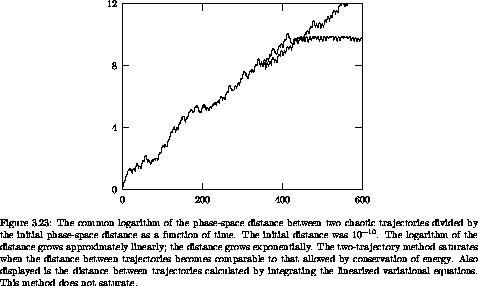On the other hand, the distance between two initially nearby regular trajectories grows much more slowly. Figure 3.24 shows the distance between two regular trajectories as a function of time. The distance grows linearly with time.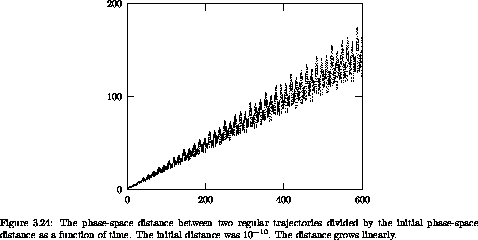It is remarkable that Hamiltonian systems have such radically different types of trajectories. On the surface of section the chaotic and regular trajectories differ in the dimension of the space that they explore. It is interesting that along with this dimensional difference there is a drastic difference in the way chaotic and regular trajectories separate. For higher dimensional systems the surface of section technique is not as useful, but trajectories are still distinguished by the way neighboring trajectories diverge: some diverge exponentially whereas others diverge approximately linearly. Exponential divergence is the hallmark of chaotic behavior.

The rate of exponential divergence is quantified by the slope of the graph of log(d(t)/d(0)). We can estimate the rate of exponential divergence of trajectories from a particular phase-space trajectory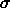by choosing a nearby trajectory' and computing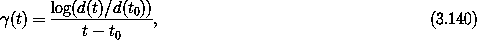where d(t) = ||'(t) -(t) ||. A problem with this ``two-trajectory'' method is illustrated in figure 3.23. For strongly chaotic trajectories two initially nearby trajectories soon find themselves as far apart as they can get. Once this happens the distance no longer grows. The estimate of the rate of divergence of trajectories is limited by this ``saturation.''

We can improve on this method by studying a variational system of equations. Let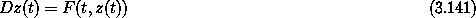be the system of equations governing the evolution of the system. A nearby trajectory z' satisfies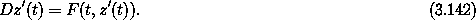The difference= z' - z between these trajectories satisfies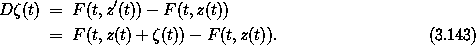Ifis small we can approximate the right-hand side by a derivative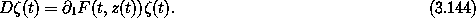This set of ordinary differential equations is called the variational equations for the system. It is linear inand driven by z.

Let d(t) = ||(t) ||; then the rate of divergence can be estimated as before. The advantage of this ``variational method'' is that(t) can become arbitrarily large and its growth still measures the divergence of nearby trajectories. We can see in figure 3.23 that the variational method gives nearly the same result as the two-trajectory method up to the point at which the two-trajectory method saturates.30

The Lyapunov exponent is defined to be the infinite time limit of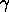(t), defined by equation (3.140), in which the distance d is computed by the variational method. Actually, for each trajectory there are many Lyapunov exponents, depending on the initial direction of the variation. For an N-dimensional system, there are N Lyapunov exponents. For a randomly chosen(t0), the subsequent growth of(t) has components that grow with each of the Lyapunov exponents. In general, however, the growth of(t) will be dominated by the largest exponent. The largest Lyapunov exponent thus can be interpreted as the typical rate of exponential divergence of nearby trajectories. The sum of the largest two Lyapunov exponents can be interpreted as the typical rate of growth of the area of two-dimensional elements. This interpretation can be extended to higher-dimensional elements: the rate of growth of volume elements is the sum of all the Lyapunov exponents.

In Hamiltonian systems, the Lyapunov exponents must satisfy constraints, which we will justify later. Lyapunov exponents come in pairs: for every Lyapunov exponent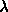its negation -is also an exponent. For every conserved quantity, one of the Lyapunov exponents is zero, as is its negation. So the Lyapunov exponents can be used to check for the existence of conserved quantities. The sum of the Lyapunov exponents for a Hamiltonian system is zero, so volume elements do not grow exponentially. We will see in the next section that phase-space volume is actually conserved for Hamiltonian systems.

30 In strongly chaotic systems(t) may become so large that the computer can no longer represent it. To prevent this we can replaceby/c whenever(t) becomes uncomfortably large. The equation governingis linear, so except for the scale change, the evolution is unchanged. Of course we have to keep track of these scale changes when computing the average growth rate. This process is called ``renormalization'' to make it sound impressive.